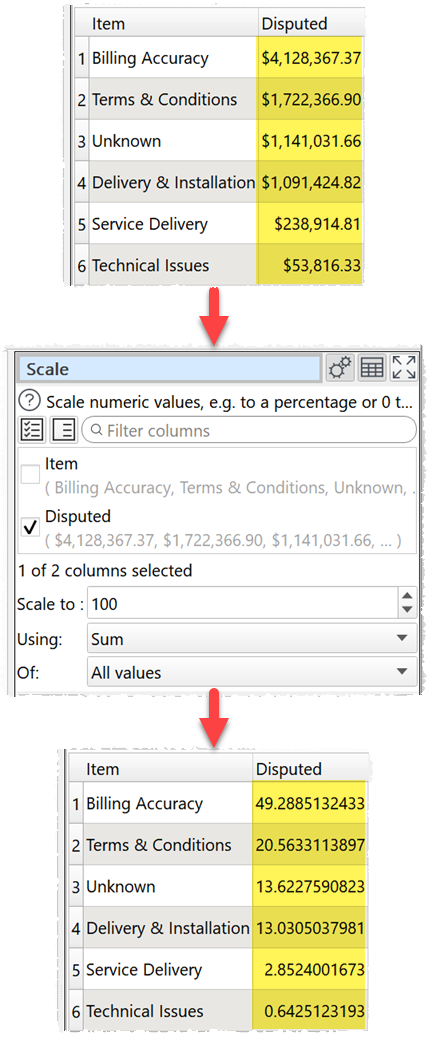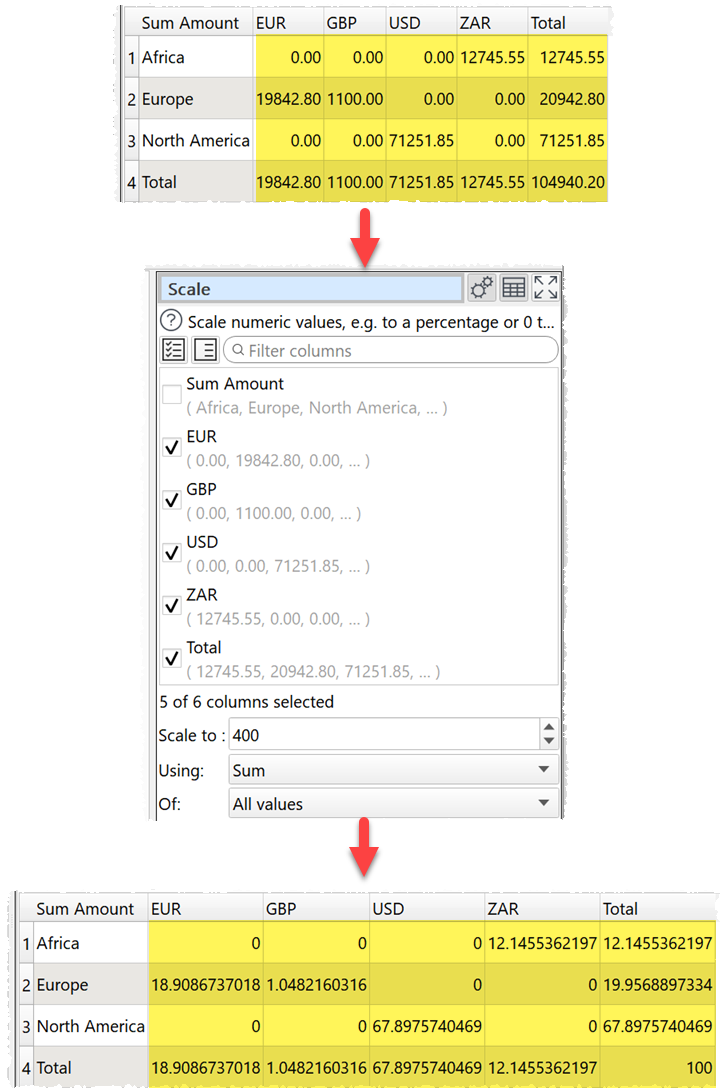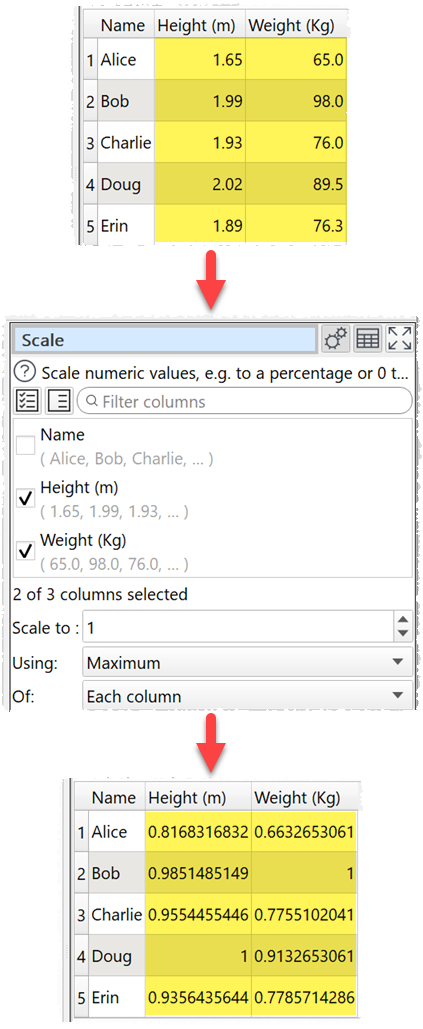﻿ Reference > Transforms > Scale

Scale

Description

Scale numeric values, e.g. to a percentage or 0 to 1.

Examples

Scale a single column to a percentage:Scale a table with row, column and grand totals to a percentage (scale to 400 as each value is also added to the row total, column total and grand total):Scale heights and weights so that the largest in each column is 1.One.

Options

Set Columns to the columns whose values you want to scale.

Set Scale to the value you want to scale to. E.g. 100 for a percentage.

Set Using depending on whether you want to use the Sum or Maximum of values for scaling.

Set Of depending on whether you want to scale for Each column, Each row or for All values.

Notes

Whether values are interpreted as numbers depends on Locale in the Preferences window.

Non-numeric values (including empty values) are ignored.

The results for negative values are undefined.

To replace empty values with zeros use the Replace transform.

To modify the numerical precision of the results use the Num Format transform.

To add a '%' at the end of values use the Insert transform.

To copy columns before using Scale use Copy Cols .

Warnings are shown in the Warnings tab for non-numeric values.